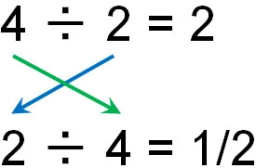# A student 4

A student knows that ¾ x 4 is the same as 4 x ¾
The student assumes that 4 ÷ ¾ is the same as ¾ ÷ 4
Is the student correct?

Result

x=

### Step-by-step explanation:Did you find an error or inaccuracy? Feel free to write us. Thank you!

Tips for related online calculators
Need help calculating sum, simplifying, or multiplying fractions? Try our fraction calculator.